# Consider this system at equilibrium. A(aq)<--->B(aq) deltaH=-750 kJ/mol What can be said about Q and K

Consider this system at equilibrium. A(aq)<—>B(aq) deltaH=-750 kJ/mol What can be said about Q and K immediately after an increase in temperature?
A) Q > K because Q increased
B) Q > K because K decreased
C) Q < K because Q decreased
D) Q < K because K increased
E) Q = K because neither charged How will the system respond to a temperature increase?
A) shift left B) shift right C) no change

Concepts and reason
The equilibrium constant (K) of the reaction gives the relation between concentration of the reactants and products. The reaction quotient is the evaluation of the arbitrary stage of the reaction.
Reaction quotient of a reaction gives the relationship between the activities of a product and activities of reactants.
The effect of temperature change on equilibrium can be explained by Le chatelier’s principle.

Fundamentals
For a reaction :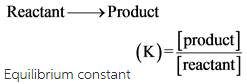The reaction quotient**(Q)** is defined in the same way as the equilibrium constant.
Then,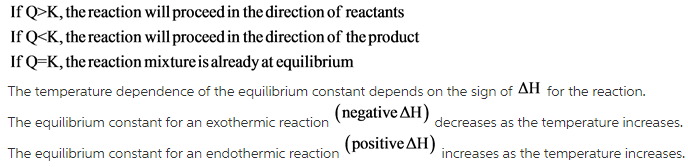Explanation: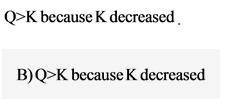Explanation:

The wrong choices are:
B) shift to the right and C) no change

Explanation:

Correct choice is A) shift left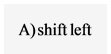Explanation:
Consider the reaction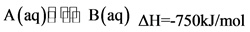Here,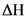is negative; it indicates that the reaction is exothermic. According to Le Chatliers principle, for an exothermic reaction, if the temperature increases, the equilibrium shifts towards the reactant side. Therefore, the equilibrium shifts to the left side.